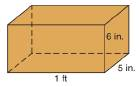Chapter 9.1, Problem 27EElementary Geometry For College St...

7th Edition
Alexander + 2 others
ISBN: 9781337614085

Solutions

Chapter
SectionElementary Geometry For College St...

7th Edition
Alexander + 2 others
ISBN: 9781337614085
Textbook Problem

The box with dimensions indicated is to be constructed of materials that cost 1 cent per square inch for the lateral surface and 2 cents per square inch for the bases. What is the total cost of constructing the box?To determine

To find:

The total cost of constructing the box.

Explanation

Given,

The dimensions of the box is,

1 ft ×5 in. ×6 in.

The cost of making the lateral surface = 1 cent per square inch.

The cost of making the bases = 2 cent per square inch.

Here, we have

l= 1 ft,

w=5 in., and

h=6 in.

Of the above three given measures, length of the box is measured by the unit of foot while the other two are measured by the unit of inches.

Thus, it is necessary to convert the measure of length from the unit of foot to inches, for the units need to be uniform and also as the cost of making is given for one square inch.

1 foot =12 in.

Thus,

l = 1ft = 12 in.

Now, find the lateral area (L) and the area of the bases of the box.

The lateral area of the box is given by the formula L=2wh+hl substute, the values of

l=12 in.

w=5 in.

h=6 in

Still sussing out bartleby?

Check out a sample textbook solution.

See a sample solution

The Solution to Your Study Problems

Bartleby provides explanations to thousands of textbook problems written by our experts, many with advanced degrees!

Get Started

Find more solutions based on key concepts